## Drop down Tab menu for Site

### FIGURE OUT PUZZLES - matchstick puzzles (01)Question 1
Move three matchsticks to correct the equation.
There's more than two solutions.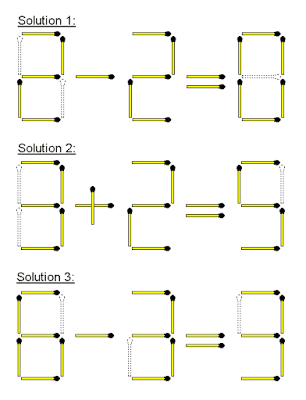Question 2
Move two matchsticks to make the equation true.
*More than one solution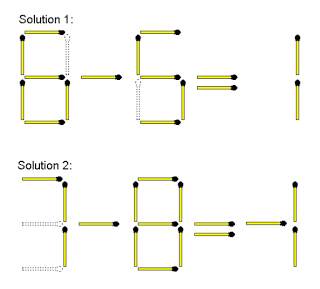Question  3
Move one matchstick to equal the number twoQuestion  4Question  5
Move only 1 matchstick to create 2 squares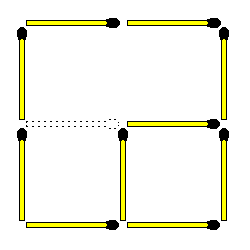Question  6
Move two matchsticks to make the equation true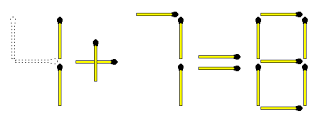Question  7
Add two matchsticks to make the equation true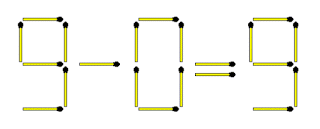Question  8
Move only 2 matchsticks to create 6 squares and 4 rectangles. The following rules apply:
.
1. Squares must be equal in size
2. Rectangles must be equal in size
3. Not all squares or rectangles have to be connected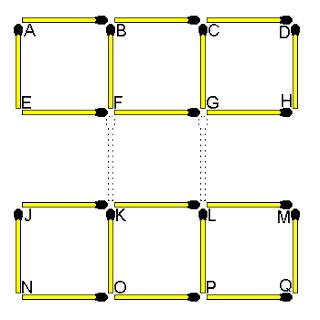Question 9
Take away two matchsticks to make the equation trueQuestion 10
Move 3 matchsticks to create 9 squares. The squares must be equal in sizeQuestion 11
What is the relationship or link between the 4 figures below?

Remove the same 2 matchsticks from each figure and turn your head to the right. It forms the letters V W X Y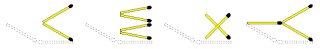Question 12
Can you decipher the hidden word in this figure by linking row 1 with row 2

By flipping row 1 and row 2 upside down and merging them togetherQuestion  13
Move two matchsticks to make the equation true.
*More than one solution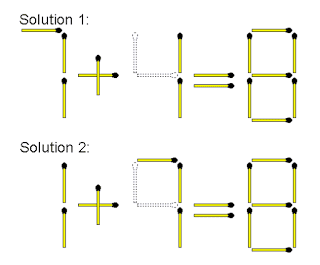Question  14
Move two matchsticks to make this equation correct.
*more than two solutionsQuestion  15
Take away only 1 matchstick to leave 4 equal squares. All the squares must be connected and all matchsticks must form part of a square.Question  16

C is the odd one out. Adding 1 matchstick to any other layout forms a capital letter.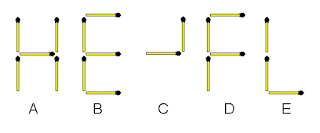Question  17
Move three matchsticks to make the equation true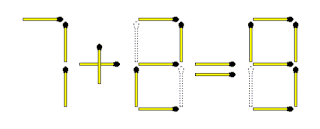Question  18
Take away two matchsticks to make the equation trueQuestion 19
How would you show that this equation is correct by just adding only one matchstick